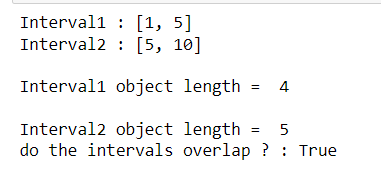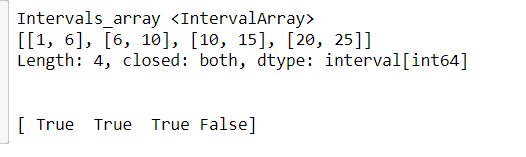GeeksforGeeks App
Open AppBrowser
Continue

# Python Pandas – Check whether two Interval objects that share closed endpoints overlap

In this article, we will cover how to check whether two intervals with sharing endpoint overlap or not. For this, we use the Interval class from pandas and the overlaps() method for all the interval-related operations.

Syntax: Interval.overlaps()

parameters:

• other : interval object. Check for an overlap using this interval.

Returns : bool . returns true if two intervals overlap. else it returns false.

## Stepwise Implementation

Step 1:

Import all the required libraries.

`import pandas`

Step 2:

Create the two Intervals that share closed endpoints

```pd.Interval(1,5, closed =both")
pd.Interval(5,10, closed="both")```

Step 3:

Check whether the intervals overlap or not using the overlaps() method. It returns a bool.

`IsOverlap = Interval1.overlaps(Interval2)`

## Code Implementations

Example 1: Create and then check if two intervals that share closed endpoints overlap.

## Python3

 `# importing pandas library``import` `pandas as pd`` ` `# Creating two closed intervals that``# share the endpoint``Interval1 ``=` `pd.Interval(``1``, ``5``, closed``=``'both'``)``Interval2 ``=` `pd.Interval(``5``, ``10``, closed``=``'both'``)`` ` `# printing the intervals``print``(``"Interval1 :"``, Interval1)``print``(``"Interval2 :"``, Interval2)`` ` `# display the length of both Interval1``# and Interval2 objects``print``(``"\nInterval1 object length = "``, Interval1.length)``print``(``"\nInterval2 object length = "``, Interval2.length)`` ` `# Check whether both the intervals overlap``print``(``"do the intervals overlap ? :"``, Interval1.overlaps(Interval2))`

Output:Example 2: Create and then check if an array of intervals that share closed endpoints overlap a given interval [3,16].

## Python3

 `# importing pandas library``import` `pandas as pd``Intervals ``=` `pd.arrays.IntervalArray.from_tuples(``    ``[(``1``, ``6``), (``6``, ``10``), (``10``, ``15``), (``20``, ``25``)], closed``=``"both"``)`` ` `# Display the IntervalArray``print``(``"Intervals_array"``, Intervals)`` ` `# check if the given intervals overlap with [3,16]``print``(Intervals.overlaps(pd.Interval(``3``, ``16``, closed``=``'both'``)))`

Output:My Personal Notes arrow_drop_up